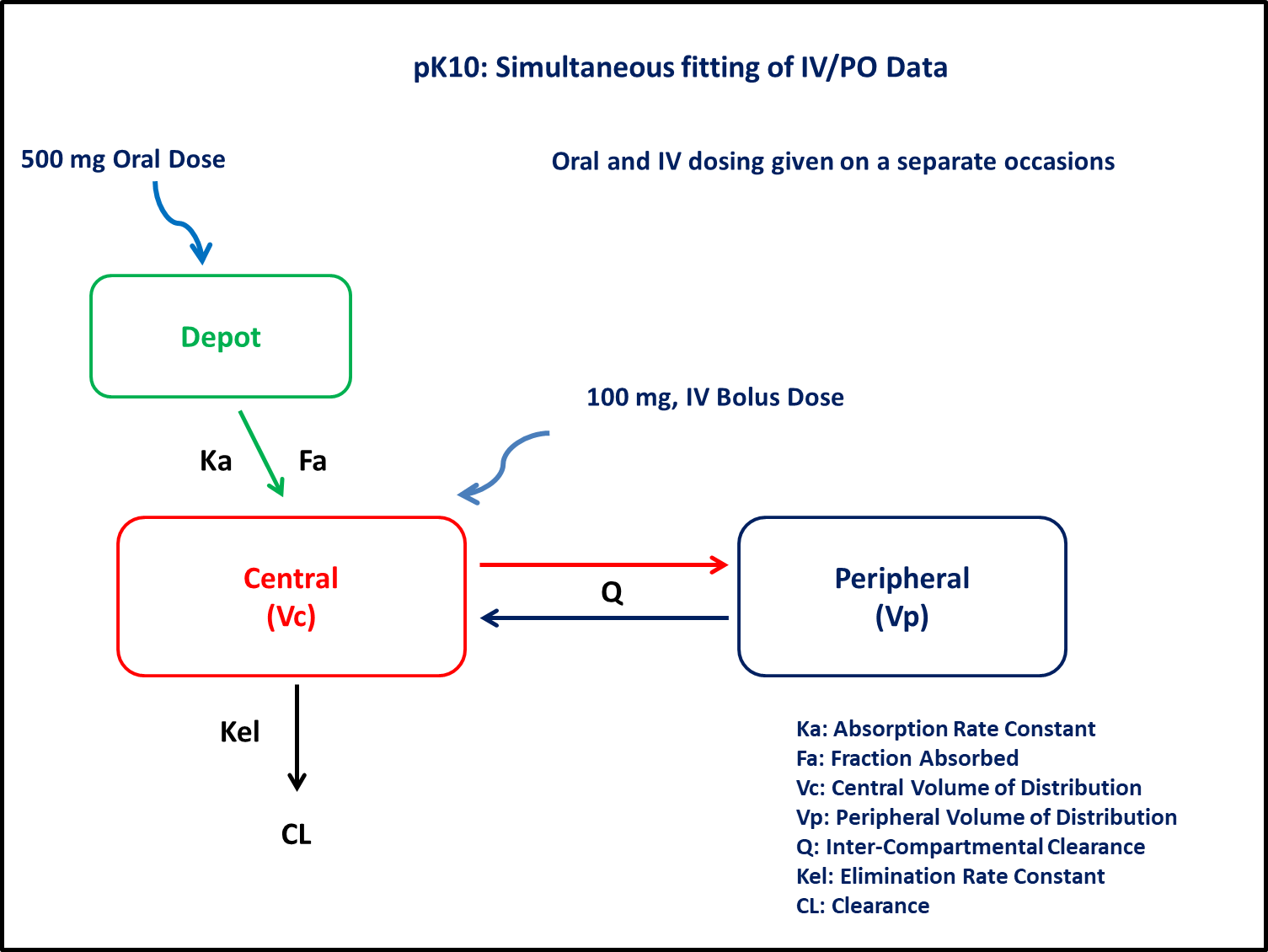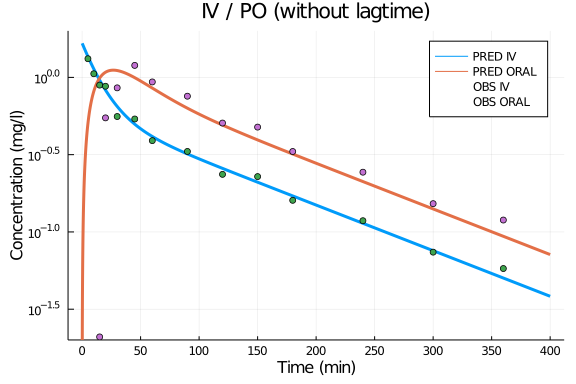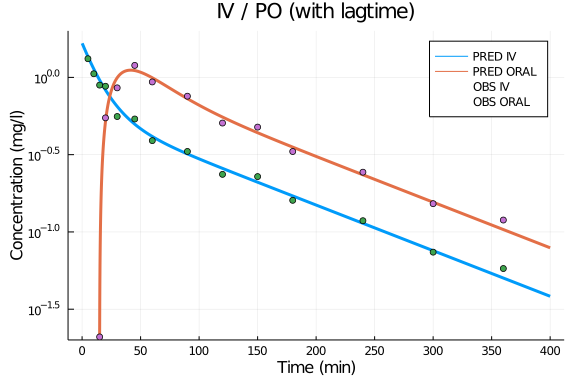# Exercise PK10 - Simultaneous fitting of IV/PO data

### Background

• Structural model - Two compartment linear elimination with first order absorption

• Route of administration - IV bolus and Oral given on separate occasions

• Dosage regimens - 100mg IV Bolus and 500mg Oral

• Subject - 1### Learning Outcome

In this model, Simultaneous fitting of iv/po data will help you to understand the disposition of drug following iv and oral (with / without lag time).

### Objectives

In this tutorial, you will learn how to build two compartment model and to simulate the model for a single subject.

### Libraries

call the "necessary" libraries to get start.

using Pumas
using Plots
using CSV
using StatsPlots
using Random


### Model

In this two compartment model, we administer dose to Depot and Central compartment.

pk_10           = @model begin
@param begin
tvvc        ∈ RealDomain(lower=0)
tvvp        ∈ RealDomain(lower=0)
tvq         ∈ RealDomain(lower=0)
tvcl        ∈ RealDomain(lower=0)
tvka        ∈ RealDomain(lower=0)
tvfa        ∈ RealDomain(lower=0)
tvlag       ∈ RealDomain(lower=0)
Ω           ∈ PDiagDomain(7)
σ²_prop     ∈ RealDomain(lower=0)
end

@random begin
η           ~ MvNormal(Ω)
end

@pre begin
Vc          = tvvc * exp(η)
Vp          = tvvp * exp(η)
Q           = tvq * exp(η)
Cl          = tvcl * exp(η)
Ka          = tvka * exp(η)
bioav       = (Depot=tvfa * exp(η),)
lags        = (Depot=tvlag * exp(η),)
end

@dynamics begin
Depot'      = -Ka*Depot
Central'    =  Ka*Depot + (Q/Vp)*Peripheral - (Q/Vc)*Central - (Cl/Vc)*Central
Peripheral' =  (Q/Vc)*Central - (Q/Vp)*Peripheral
end

@derived begin
cp          = @. Central/Vc
dv          ~ @. Normal(cp, sqrt(cp^2*σ²_prop))
end
end

PumasModel
Parameters: tvvc, tvvp, tvq, tvcl, tvka, tvfa, tvlag, Ω, σ²_prop
Random effects: η
Covariates:
Dynamical variables: Depot, Central, Peripheral
Derived: cp, dv
Observed: cp, dv


### Parameters

Parameters provided for simulation. tv represents the typical value for parameters.

• Vc - Volume of Central Compartment (L)

• Vp - Volume of Peripheral Compartment (L)

• Q - InterCompartmental clearance (L/min)

• Cl - Clearance from Central InterCompartmental (L/min)

• Ka - Absorption rate constant (min⁻¹)

• Fa - Fraction of drug absorbed

• lags - Lagtime (min)

• Ω - Between Subject Variability

• σ - Residual error

##### IV / PO - without lagtime
param1 = ( tvvc    = 59.9348,
tvvp    = 60.5898,
tvq     = 1.55421,
tvcl    = 0.967573,
tvka    = 0.0471557,
tvfa    = 0.318748,
tvlag   = 0,
Ω       = Diagonal([0.0,0.0,0.0,0.0,0.0,0.0,0.0,0.0]),
σ²_prop = 0.01)

(tvvc = 59.9348, tvvp = 60.5898, tvq = 1.55421, tvcl = 0.967573, tvka = 0.0
471557, tvfa = 0.318748, tvlag = 0, Ω = [0.0 0.0 … 0.0 0.0; 0.0 0.0 … 0.0 0
.0; … ; 0.0 0.0 … 0.0 0.0; 0.0 0.0 … 0.0 0.0], σ²_prop = 0.01)

##### IV / PO - with lagtime
param2 = ( tvvc    = 59.9348,
tvvp    = 60.5898,
tvq     = 1.55421,
tvcl    = 0.967573,
tvka    = 0.0471557,
tvfa    = 0.318748,
tvlag   = 14.8187,
Ω       = Diagonal([0.0,0.0,0.0,0.0,0.0,0.0,0.0,0.0]),
σ²_prop = 0.01)

(tvvc = 59.9348, tvvp = 60.5898, tvq = 1.55421, tvcl = 0.967573, tvka = 0.0
471557, tvfa = 0.318748, tvlag = 14.8187, Ω = [0.0 0.0 … 0.0 0.0; 0.0 0.0 …
0.0 0.0; … ; 0.0 0.0 … 0.0 0.0; 0.0 0.0 … 0.0 0.0], σ²_prop = 0.01)


### DosageRegimen

DosageRegimen - Single Subject receiving 100mg Intravenous Bolus dose and 500mg Oral dose on different occasion.

##### IV
ev1     = DosageRegimen(100,time=0,cmt=2)
sub1_iv = Subject(id=1,events=ev1)

Subject
ID: 1
Events: 1

##### PO
ev2     = DosageRegimen(500,time=0,cmt=1)
sub1_po = Subject(id=1,events=ev2)

Subject
ID: 1
Events: 1


### Simulation

##### IV

Lets simulate plasma concentration with specific observation times after IV bolus.

Random.seed!(123)
sim_iv_sub1 = simobs(pk_10, sub1_iv, param1, obstimes=0.1:0.1:400)
df_iv       = DataFrame(sim_iv_sub1)

##### PO

Lets simulate plasma concentration with specific observation times after PO (with/without lagtime)

Random.seed!(123)
sim_po_sub1_nolag = simobs(pk_10, sub1_po, param1, obstimes=0.1:0.1:400)
df_po_nolag       = DataFrame(sim_po_sub1_nolag)
Random.seed!(123)
sim_po_sub1_lag   = simobs(pk_10, sub1_po, param2, obstimes=0.1:0.1:400)
df_po_withlag     = DataFrame(sim_po_sub1_lag)


### DataFrame & Plot

Use the dataframe for plotting

obs_iv         = filter(x -> x.time in [0,5, 10, 15, 20, 30, 45, 60, 90, 120, 150, 180, 240, 300, 360], df_iv)
obs_po_nolag   = filter(x -> x.time in [0,5, 10, 15, 20, 30, 45, 60, 90, 120, 150, 180, 240, 300, 360], df_po_nolag )
obs_po_withlag = filter(x -> x.time in [0,15, 20, 30, 45, 60, 90, 120, 150, 180, 240, 300, 360], df_po_withlag)

#without lagtime
@df df_iv plot(:time, :cp, yaxis=:log,
label="PRED IV",title="IV / PO (without lagtime)", xlabel="Time (min)", ylabel="Concentration (mg/l)",
xticks=[0,50,100,150,200,250,300,350,400], ylims=(0.02,2), lw=3)
@df df_po_nolag plot!(:time, :cp, label="PRED ORAL", lw=3)
@df obs_iv scatter!(:time, :dv, label="OBS IV")
@df obs_po_withlag scatter!(:time, :dv, label="OBS ORAL")#with Lagtime
@df df_iv plot(:time, :cp, yaxis=:log,
label="PRED IV",title="IV / PO (with lagtime)", xlabel="Time (min)", ylabel="Concentration (mg/l)",
xticks=[0,50,100,150,200,250,300,350,400], ylims=(0.02,2), lw=3)
@df df_po_withlag plot!(:time, :cp, label="PRED ORAL", lw=3)
@df obs_iv scatter!(:time, :dv, label="OBS IV")
@df obs_po_withlag scatter!(:time, :dv, label="OBS ORAL")